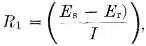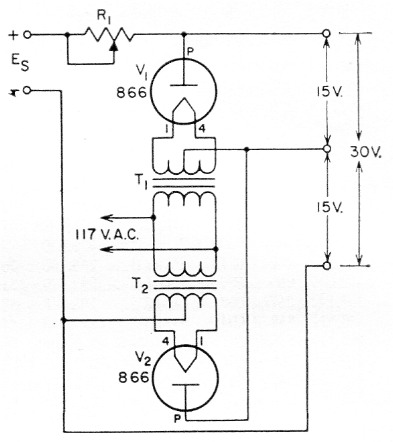# Stable low voltage supply

Home - Techniek - Electronica - Radiotechniek - Radio amateur bladen - QST - Stable low voltage supply

Here is a low voltage power supply that can be used for powering transistor circuits, for bias, or for any job that requires a stable low voltage. When using the unit as a bias supply, R1 in Fig. 1 is adjusted without transmitter amplifier excitation until V1 draws about 5 mA. The value of R1 can be calculated by the formula:where Es is the source voltage, Er is the rated voltage drop across the tube (15 volts for the 866), and I is the current. Additional voltage to bring the bias up to the operating value when excitation is applied can be obtained from a grid leak resistor. When an 866 tube is used in the circuit, a maximum current of 250 mA may be obtained at the low voltage output. Other tubes, such as the 816 or 83 can also be used and more can be added in series as shown in the diagram to give steps of approximately 15 volts each. Notice that filament power must be supplied by separate transformers, T1 and T2.Fig. 1. Stable low voltage power supply. Voltage (Es) for the circuit can be supplied from any available source.

When using this circuit for a bias supply, the power source (E8) should have its positive terminal grounded and R1 inserted in the negative lead.

If the supply is used as a general-use low voltage supply, R1 is adjusted until the plate current of the tube is at maximum (250 mA for the 866).

Capt. A.B. Jones, K9LKC.